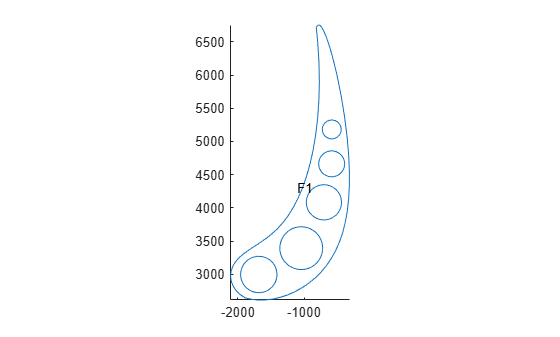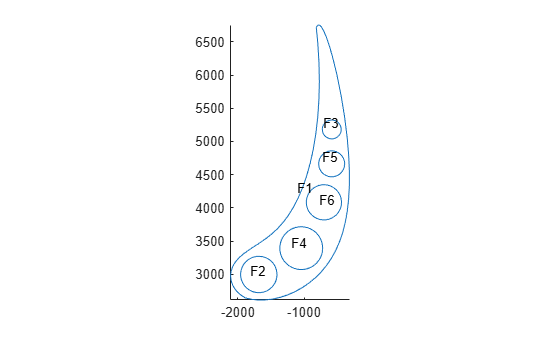# 3-D Multidomain Geometry from 2-D Geometry

This example shows how to create a 3-D multidomain geometry by extruding a 2-D geometry imported from STL data. The original 2-D geometry represents a cooled turbine blade section defined by a 2-D profile.

Before extruding the geometry, this example modifies the original 2-D profile as follows:

• Translates the geometry to move the tip to the origin

• Aligns the chord with the x-axis

• Changes the dimensions from inches to millimeters

First, create a PDE model.

`model = createpde;`

Import the geometry into the model.

`g = importGeometry(model,"CooledBlade2D.STL");`

Plot the geometry with the face labels.

```figure pdegplot(model,"FaceLabels","on")```Translate the geometry to align the tip of the blade with the origin.

```tip = [1.802091,-127.98192215]; translate(g,tip);```

Rotate the geometry to align the chord with the x-axis.

```angle = -36.26005; rotate(g,angle);```

Scale the geometry to convert from inches to millimeters.

`scale(g,[25.4 -25.4]);`

Plot the resulting geometry with the face labels.

```figure pdegplot(model,"FaceLabels","on")```Fill the void regions with faces and plot the resulting geometry.

```g = addFace(g,{3, 4, 5, 6, 7}); figure pdegplot(model,"FaceLabels","on")```Extrude the geometry to create a stacked multilayer 3-D model of the blade. The thickness of each layer is 200 mm.

`g = extrude(g,[200 200 200]);`

Plot the geometry with the cell labels.

```figure pdegplot(model,"CellLabels","on","FaceAlpha",0.5)```